Question-and-Answer Resource for the Building Energy Modeling Community
Get started with the Help page

# Water Coil Sizing, heating and coolingHi! I have a problem with water coil sizing. I set the following parameters for cooling and heating: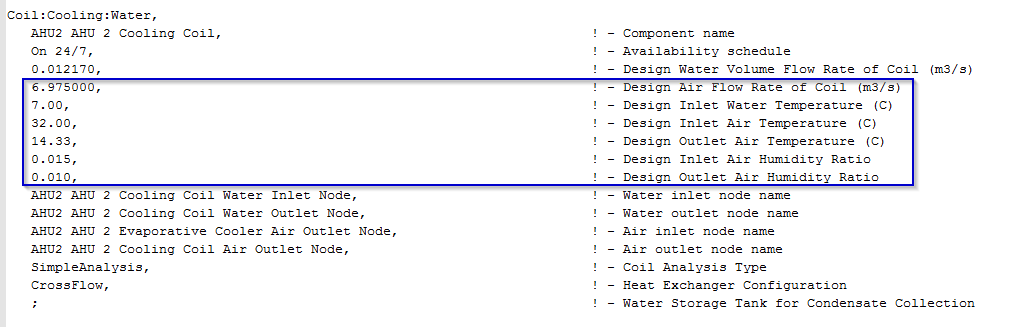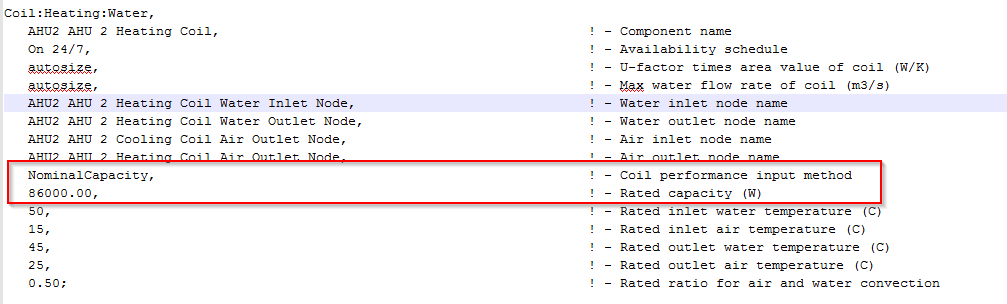Therefore, through the formula (cooling):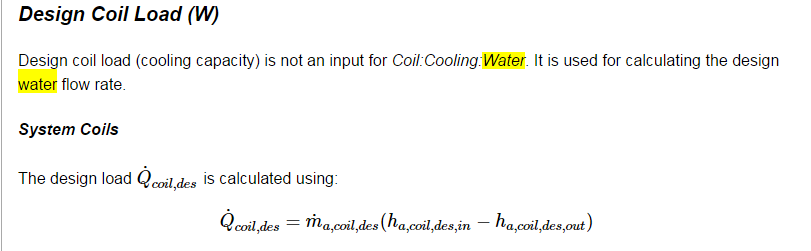I would expect to find (cooling): COOLING COIL = v[m3/s]p[kg/m3](hin-hout)[kJ/kg]=258 kW with hin=70.573 kJ/kg hout=39.685 kJ/kg

I would expect to find (heating): HEATING COIL = 86 kW

But I find this for cooling: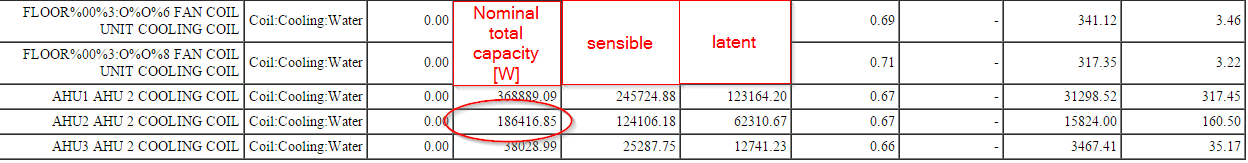and this for heating: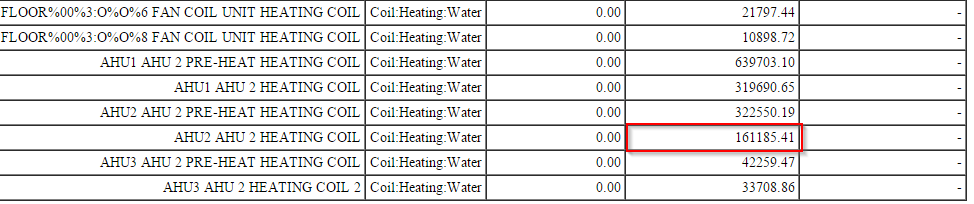How is it possible? Where did I make the mistake?

Thanks a lot

edit retag close merge delete

I assumed as constant the air density as 1,2 kg/m3 but it donsn't make that difference

Sort by » oldest newest most voted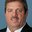You are correct up to a point. The water coil model does result in a design capacity very near your calculations. However, that capacity is only used to calculate a coil UA value.

Hair,in = 70561.3
Hair,out=39675.1
Qcoil,des = 259107.0


Other coil parameters are also calculated during this sizing effort, such as bypass factor, SHR, etc. The model then changes the inlet conditions to nominal values to determine what physical coil would be used for these application input parameters (i.e., the user entered coil conditions shown above).

Tcoil,inlet,air,db = 26.67
Tcoil,inlet,air,wb = 19.44
Tcoil,inlet,water = 6.67
Qcoil,actual = 186127.2


These calculations result in the following:

Tcoil,outlet,air = 11.96
Wcoil,outlet,air = 0.0084
Tcoil,outlet,water = 10.32


Realize now that your coil inlet air temperature was 32 C. This physical water coil would have a much higher capacity if the inlet air temperature was increased from 26.7 C to 32 C. The result is the actual physical coil you would specify for your application (i.e., coil area, etc.)

more

Thanks a lot! Just to be sure I understood right: does it mean that the sizing is correct but in the report is given with different reference values? I'm modelling an AHU from its technical specifications so I wanted to be sure about it.

You coil is sized correctly according to your inputs. I would check the results using report variables for node temperatures before and after the coil to assure things are working correctly.

Perfect, you helped me a lot.. again!. Thank you very much for all these advices

I had the same problem in another model but this time this is causing a lot of problems and unmet hours. Is there a way to force the cooling coil sizing? I want E+ to size the coil exactly as it has been designed

@rraustad : I thought it was just a report difference. In this new case I have a fan coil cooling water coil. My input correspond to 11000 W but I find 3000 W in the report and also in the simulations so that the mean air temperature is always over the setpoint